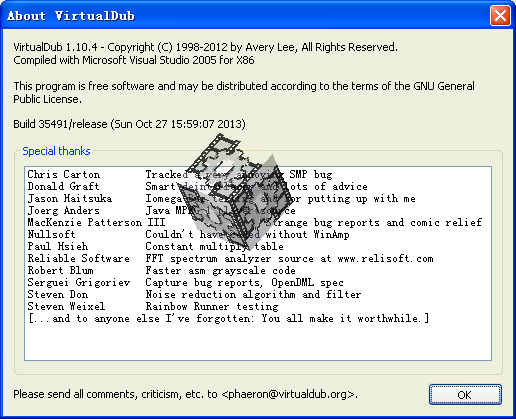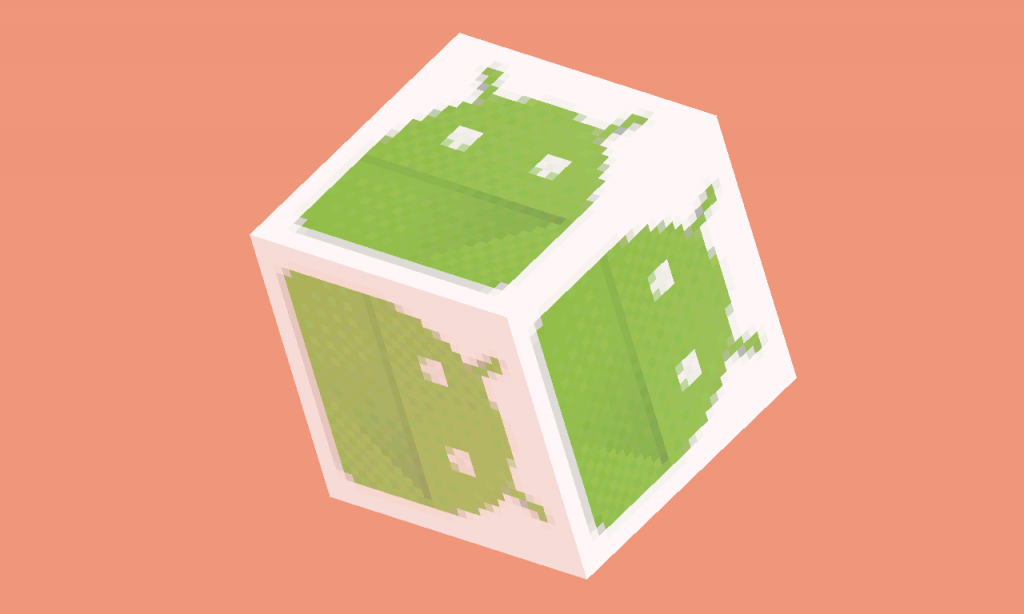# Android: 直接在bitmap上绘制一个立方体• 移植相关代码到Android中

1. 获取cubic表面的纹理图片，这里使用default application icon
2. 当view size changed的时候，更新绘制cubic所使用bitmap大小，以及通知native当前窗口的大小
3. 设置定时器，每40ms更新一次

1. 更新bitmap， 使用CPU绘制cubic的每个面（纹理贴图）

```extern "C" void Java_com_brobwind_cubic_CubicActivity_setBounce(JNIEnv * /*env*/, jobject,
jint left, jint top, jint right, jint bottom)
{
// Initialize cube vertices.
int i;
float rs;
RECT r = RECT(left, top, right, bottom);

if (r.right > r.bottom)
rs = r.bottom / (3.6f*1.0f);
else
rs = r.right / (3.6f*1.0f);

...
}
```• 关于RGB_565 bitmap进行alpha blending

```void RenderTriangle(Pixel16 *dst, long dstpitch, Pixel16 *tex, TriPt *pt1, TriPt *pt2, TriPt *pt3, uint32_t s) {
...
while(x1 < x2) {
uint32_t A = tex[(u>>27) + (v>>27)*32];
uint32_t B = dst[x1];

#ifdef RGB_1555
uint32_t p0 = (((A^B ) & 0x7bde)>>1) + (A&B);
uint32_t p  = (((A^p0) & 0x7bde)>>1) + (A&B);
dst[x1] = (uint16_t)(((((p&0x007c1f)*s + 0x004010) & 0x0f83e0) + (((p&0x0003e0)*s + 0x000200) & 0x007c00)) >> 5);
#else // RGB_565
dst[x1] = blend565(B, A, s);
#endif
++x1;

u += dudxi;
v += dvdxi;
}
...
}```

Alpha blending without SIMD support

Now that we‘ve covered averaging bitfields, how to efficiently alpha blend with a factor other than one-half?

Alpha blending is normally done using an operation known as linear interpolation, or lerp:

lerp(a, b, f) = a*(1-f) + b*f = a + (b-a)*f

…where a and b are the values to be blended, and f is a blend factor from 0-1 where 0 gives a and 1 gives b. To blend a packed pixel, you could just expand all channels of the source and destination pixels and do the blend in floating point, but it really hurts to see this, since the code turns out nasty on practically any platform. Unless you‘ve got a platform that gets pixels in and out of a floating-point vector really easily, you should use integer math for fast alpha blending.

So how to blend quickly without resorting to per-channel?

First, if you are dealing with an alpha channel instead of a constant alpha value, chances are that the alpha value ranges from 0 to 2^N-1, which is not a convenient factor for division. You could cheat and just divide by 2^N, but that leads to the unpleasant result of either the fully transparent or opaque case not working correctly (sloppy). Conditionally adding one to the alpha value fixes this at the cost of introducing a tiny amount of error. I used to add the high bit, thus mapping [128,255] to [129,256] for 8-bit values; I‘m told that shifting [1,255] to [2,256] leads to better accuracy. Either way will prevent the glaring error cases, though.

The next step is to reformulate the blend equation in terms of integer math:

lerp(a, b, f) = a + (((b-a) * f + round) >> shift)

where round = 1 << (shift1).

To eliminate some of the pack/unpack work, realize that you can alpha blend a channel in place as long as you isolate it and have enough headroom above it in the machine word to accommodate the intermediate result of the multiply. In other words, instead of extracting red = (pixel >> 16)&0xff, blending that, and then shifting it back up, simply blend (pixel & 0x00ff0000).

Now, the magic: you can actually do more than one bitfield this way as long as you have enough space between them. If you have two non-overlapping bitfields combined as (a << shift1) + (b << shift2), multiplying their combined form by an integer gives the same result as splitting them apart, multiplying each, and then recombining. For a 565 pixel, you could thus blend red and blue in the following manner (remember that the red/green/blue masks for 565 are 0xf800, 0x07e0, and 0x001f, respectively):

rbsrc = src & 0xf81f

rbdst = dst & 0xf81f

rbout = ((rbsrc * f + rbdst * (32-f) + 0x8010) >> 5) & 0xf81f

Which leads to the surprising result that you can safely subtract the two bitfields together and scale the difference without any fancy SIMD bitfield support.

rbout = (rbdst + (((rbsrc – rbdst) * f + 0x8010) >> 5)) & 0xf81f

The remaining green channel is easy. Doing it this way does limit precision in the blend factor, since you‘re limited to the number of bits of headroom you have, but five bits for 565 is decent. If you also have an alpha channel to blend, you can do so, although you might need to temporarily shift down green and alpha together to make headroom at the top of the machine word if you’re dealing with a big pixel.

What if you didn‘t have a hardware multiply, or the one you have is very slow? Well, you might use lookup tables, then. Ideally, though, you’d like to avoid inserting and extracting the channels again. One dirty trick you can use revolves around the fact that you can distribute the multiplication over the additive nature of bits, thus allowing the lookup tables to be indexed off the raw bytes instead of the channels:

unsigned blend565;

void init() {

for(unsigned alpha=0; alpha<=32; ++alpha) {

unsigned f = alpha;

for(unsigned i=0; i<256; ++i) {

blend565[alpha][i] = (((i & 0xf8)*f) << 19) + (((i & 0x07)*f) <<  3) + (0x04008010 >> 1);

blend565[alpha][i] = (((i & 0xe0)*f) >>  5) + (((i & 0x1f)*f) << 11);

}

}

}

void blend565(unsigned dst, unsigned src, unsigned alpha) {

unsigned ialpha = 32-alpha;

unsigned sum = blend565tab[alpha][src & 0xff] + blend565tab[ialpha][dst & 0xff] + blend565tab[alpha][src >> 8] + blend565tab[ialpha][dst >> 8];

sum &= 0xf81f07e0;

return (sum & 0xffff) + (sum >> 16);

}

It may look odd because we‘re actually splitting the green bitfield between the two lookup tables, but it works — essentially, it’s combining partial products from the lower and upper halves of the green bitfield. I‘ve also thrown the rounding constant into the tables to save an addition. The table’s rather big at 67K, but if you are doing alpha blending off of a constant, you can cache pointers to the two pertinent rows and then only 4K of tables are used, which is much nicer on the cache. The shifting/masking in the table lookups are also unnecessary if you load the source pixels as pairs of bytes instead of as words.

Incidentally, if you think about it, this trick can also be used to convert any bitfield-based 16-bit packed pixel format to any other bitfield-based pixel format up to 32 bits with a single routine, just by changing 2K of tables. This generally isn‘t worthwhile if you have a SIMD multiplier — Intel’s MMX application notes describe how you can abuse MMX‘s pmaddwd instruction to convert 8888 to 565 at about 2.1 clocks/pixel — but it can be handy if you find yourself without a hardware multiplier or even a barrel shifter.

1. http://www.virtualdub.org/
2. http://www.virtualdub.org/blog/pivot/entry.php?id=117
3. http://chrishecker.com/Miscellaneous_Technical_Articles